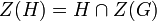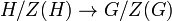# Subgroup whose center is contained in the center of the whole group

This article defines a subgroup property: a property that can be evaluated to true/false given a group and a subgroup thereof, invariant under subgroup equivalence. View a complete list of subgroup properties[SHOW MORE]

## Definition

The notion of subgroup whose center is contained in the center of the whole group has the following equivalent definitions:

No. Shorthand A subgroup of a group is termed a subgroup whose center is contained in the center of the whole group if ... A subgroup$H$ of a group$G$ is termed a subgroup whose center is contained in the center of the whole group if ...
1 center containment the center of the subgroup is contained in the center of the whole group.$Z(H) \le Z(G)$ where$Z(H)$ denotes the center of$H$ and$Z(G)$ denotes the center of$G$.
2 center equals intersection with center the center of the subgroup equals the intersection of the subgroup with the center of the whole group.$Z(H) = H \cap Z(G)$ where$Z(H)$ denotes the center of$H$ and$Z(G)$ denotes the center of$G$.
3 intersection with center equals intersection with centralizer the intersection of the subgroup with the center of the whole group equals the intersection of the subgroup with its centralizer.$H \cap C_G(H) = H \cap Z(G)$ where$C_G(H)$ is the centralizer of$H$ in$G$ and$Z(G)$ is the center of$G$.
4 inclusion induces well-defined map on inner automorphism groups the inclusion of the subgroup in the whole group induces a homomorphism of the inner automorphism groups (in fact, this homomorphism must be injective). the subgroup inclusion$H \to G$ induces a well-defined map$H/Z(H) \to G/Z(G)$, or in other words, a well-defined map$\operatorname{Inn}(H) \to \operatorname{Inn}(G)$.
This definition is presented using a tabular format. |View all pages with definitions in tabular format

## Examples

### Extreme examples

• The trivial subgroup in any group satisfies this property.
• Every group satisfies this property in itself.

### High occurrence examples

• If the whole group is an abelian group, every subgroup satisfies the property.
• A centerless group satisfies this property as a subgroup in any bigger group.

### Subgroups satisfying the property

Here are some examples of subgroups in basic/important groups satisfying the property:

Here are some examples of subgroups in relatively less basic/important groups satisfying the property:

Here are some examples of subgroups in even more complicated/less basic groups satisfying the property:

### Subgroups dissatisfying the property

Here are some examples of subgroups in basic/important groups not satisfying the property:

Here are some some examples of subgroups in relatively less basic/important groups not satisfying the property:

Here are some examples of subgroups in even more complicated/less basic groups not satisfying the property:

## Metaproperties

Metaproperty Satisfied? Proof Statement with symbols
transitive subgroup property Yes (link pending) If$H \le K \le G$ are groups such that$H$ satisfies this property in$K$ and$K$ satisfies this property in$G$, then$H$ satisfies this property in$G$.
trim subgroup property Yes In any group$G$, the whole group and the trivial subgroup satisfy this property.

## Relation with other properties

### Stronger properties

Property Meaning Proof of implication Proof of strictness (reverse implication failure) Intermediate notions
normal subgroup whose center is contained in the center of the whole group also a normal subgroup
center-fixing automorphism-balanced subgroup any center-fixing automorphism of the whole group restricts to a center-fixing automorphism of the subgroup Normal subgroup whose center is contained in the center of the whole group|FULL LIST, MORE INFO
conjugacy-closed normal subgroup normal and any two elements of the subgroup that are conjugate in the whole group are conjugate in the subgroup. (via normal subgroup whose center is contained in the center of the whole group) Normal subgroup whose center is contained in the center of the whole group|FULL LIST, MORE INFO
center-fixing automorphism-balanced subgroup
central factor product with centralizer is whole group (via conjugacy-closed normal subgroup) (via conjugacy-closed normal subgroup) Normal subgroup whose center is contained in the center of the whole group|FULL LIST, MORE INFO
direct factor factor in an internal direct product (via central factor) (via central factor) |FULL LIST, MORE INFO
central subgroup contained in the center (via central factor) (via central factor) Normal subgroup whose center is contained in the center of the whole group|FULL LIST, MORE INFO
centralizer-free subgroup its centralizer is a trivial subgroup |FULL LIST, MORE INFO
subgroup that is a centerless group its own center is trivial.